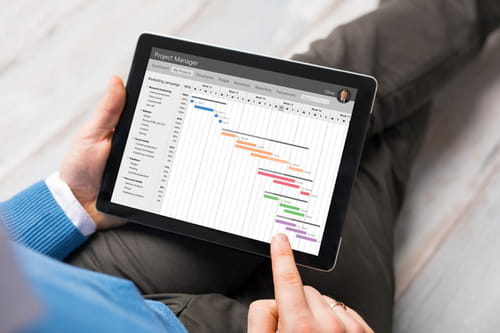2
Thanks

A few words of thanks would be greatly appreciated.

# Transfer Data From One Excel Sheet to Another Using VBA Code

In this article, we will provide a VBA code to transfer data from one Excel sheet to multiple others while respecting specific conditions.## How to Transfer Data From One Excel Sheet to Another

You can use following codes

`Sub SplitSheets() Dim DataSht, wsCrit, SplitSht As Worksheet Dim lrUnq, lrData, i As Long Dim FtrVal As String Application.ScreenUpdating = False Set DataSht = Worksheets("sheet1") 'change it to the name of your raw data sheet lrData = DataSht.Range("a" & Rows.Count).End(xlUp).Row Set wsCrit = Worksheets.Add DataSht.Range("B1:l" & lrData).AdvancedFilter Action:=xlFilterCopy, _ CopyToRange:=wsCrit.Range("A1"), Unique:=True lrUnq = wsCrit.Range("a" & Rows.Count).End(xlUp).Row For i = 2 To lrUnq FtrVal = wsCrit.Range("A" & i).Value Set SplitSht = Worksheets.Add DataSht.Select 'DataSht.ShowAllData ActiveSheet.AutoFilterMode = False ActiveSheet.Range("A1:Z" & lrData).AutoFilter Field:=2, Criteria1:=FtrVal Range("a1").Select Range(Selection, Selection.End(xlToRight)).Select Range(Selection, Selection.End(xlDown)).Select Selection.Copy SplitSht.Select Range("A1").Select ActiveSheet.Paste 'Cells.Select Cells.EntireColumn.AutoFit SplitSht.Name = FtrVal Application.CutCopyMode = False Next i Application.DisplayAlerts = False wsCrit.Delete Application.DisplayAlerts = True .AutoFilterMode = False End Sub`